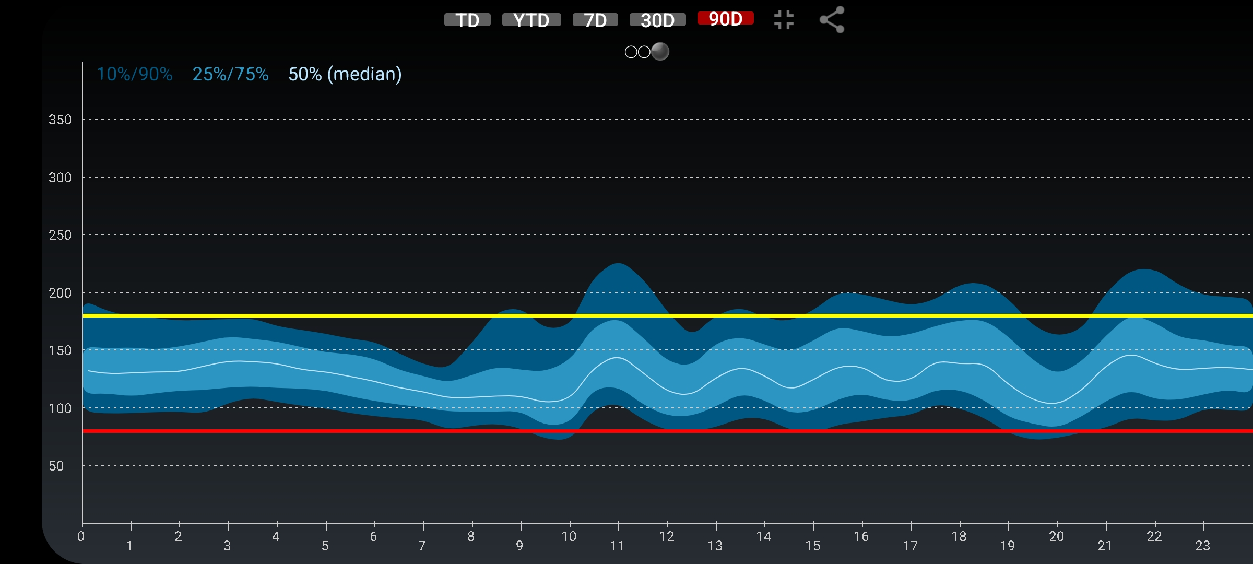# Statistics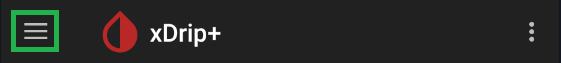Statistics view offers 3 basic statistics displays you can configure for different periods of time.

1. First select the period of time you want to calculate statistics on. ToDay, YestTerDay, last week, month or quarter.
2. You can display the graph full screen (touch it again to exit full screen mode).
3. You can share the screen as a screenshot.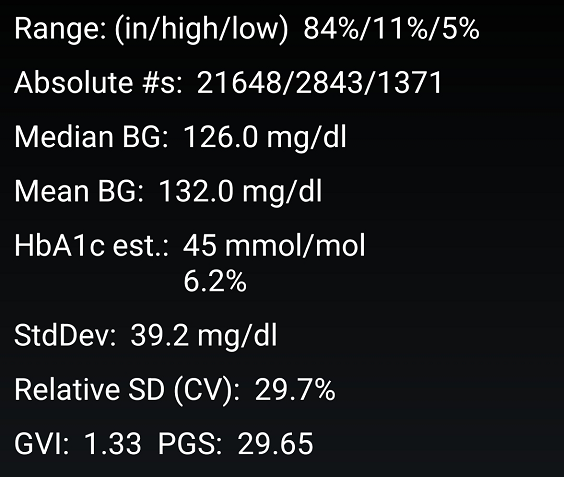Percentage in range is calculated using low and high values range defined in Menu - Glucose Units.
Absolute numbers counts the number of measurements in range, above and below.
Median BG is the central value of your data: same amount of measurements above and same below.
Mean BG is the mathematical average of all measurements.
Estimated HbA1c is only a mathematical calculation based on the average BG, and converted in mmol/mol or percent.
StdDev or standard deviation is the mathematical result of the calculation. It shows the dispersion of your BG.
Relative SD (CV) is the StdDev divided by mean and also shows the dispersion of your measurements.
GVI and PGS were parameters introduced by Dexcom see here.

Look here for explanations of these parameters, and remember they are based on measurements and depend on their quality. Bad measurements (inaccurate) will lead to bad statistics, should it be because of the sensor accuracy or your calibrations.

Swipe towards the left to see a graphical representation of your percentage in range.
Green is in range, yellow above and red below target.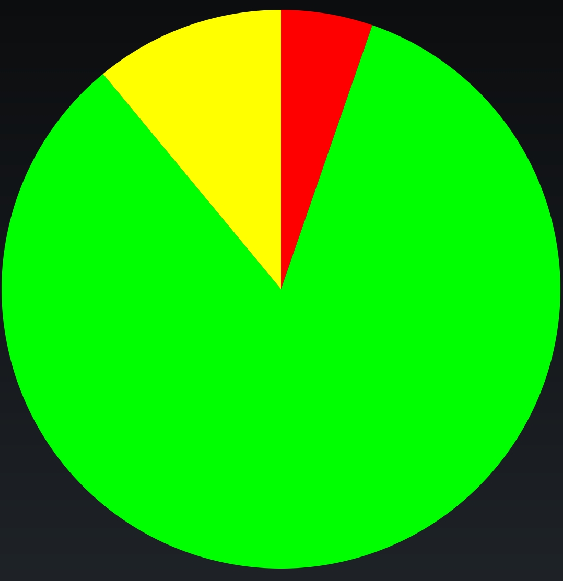Swipe again to see the percentile chart.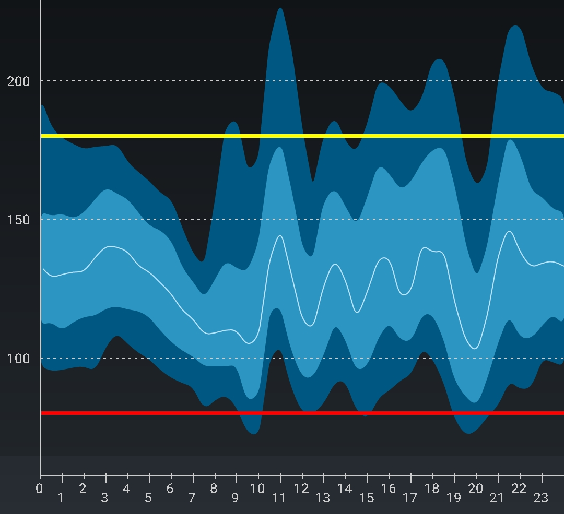Rotate the screen to make it look better.